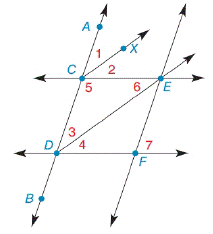Chapter 2.1, Problem 23E### Elementary Geometry For College St...

7th Edition
Alexander + 2 others
ISBN: 9781337614085

#### Solutions

Chapter
Section### Elementary Geometry For College St...

7th Edition
Alexander + 2 others
ISBN: 9781337614085
Textbook Problem

# Given: C E ↔ ∥ D F ↔ Transversal A B ↔ C X ↔ bisects ∠ A C E D E ↔ bisects ∠ C D F Prove: ∠ 1 ≅ ∠ 3To determine

To check:

Whether 1 is congruent to 3 or not.

Explanation

Given:

The given statement is,

CEDF, AB is transversal, CX bisects ACE, and DE bisects CDF.

Figure (1)

Properties used:

(1) If two parallel lines are cut by a transversal, then the pair of corresponding angles are congruent.

(2) Angle bisectors of two congruent angles bisect the angle into four congruent angles.

Approach:

The proof of the given statement is shown in the following table:

 Proof Statements Reasons 1. CE↔∥DF↔ 1. Given 2. AB↔ is transversal 2. Given. 3. ∠ACE≅∠CDF 3. If two ∥ are cut by transversal, then the pair of corresponding ∠ s are congruent 4

### Still sussing out bartleby?

Check out a sample textbook solution.

See a sample solution

#### The Solution to Your Study Problems

Bartleby provides explanations to thousands of textbook problems written by our experts, many with advanced degrees!

Get Started

## Additional Math Solutions

#### Find more solutions based on key concepts